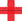top of page

## Video ResourcesIn this video, Luke or Lindsey takes students through a full Stats Medic EFFL lesson.

# Conditional Probabilities and Tree Diagrams

##### Learning Targets
• Use the general multiplication rule to calculate probabilities.

• Use a tree diagram to model a chance process involving a sequence of outcomes and to calculate probabilities.

• When appropriate, use the multiplication rule for independent events to calculate probabilities.

bottom of page